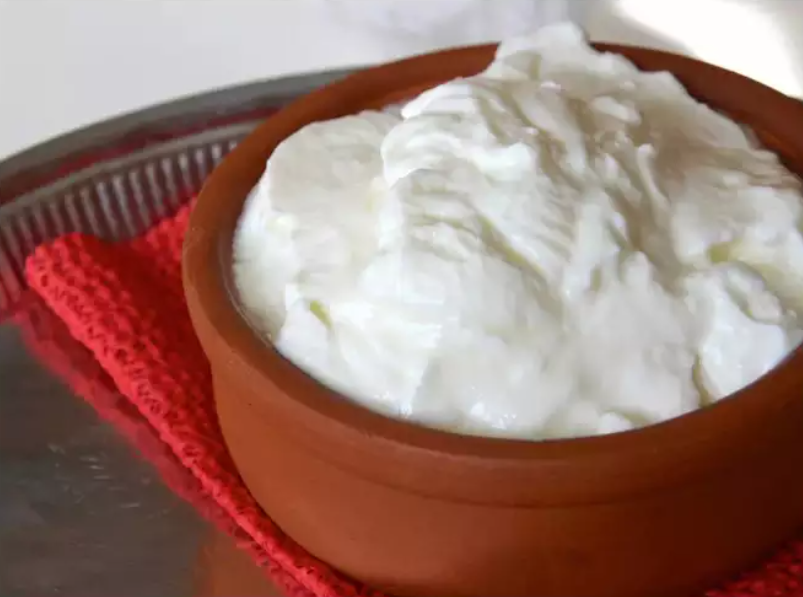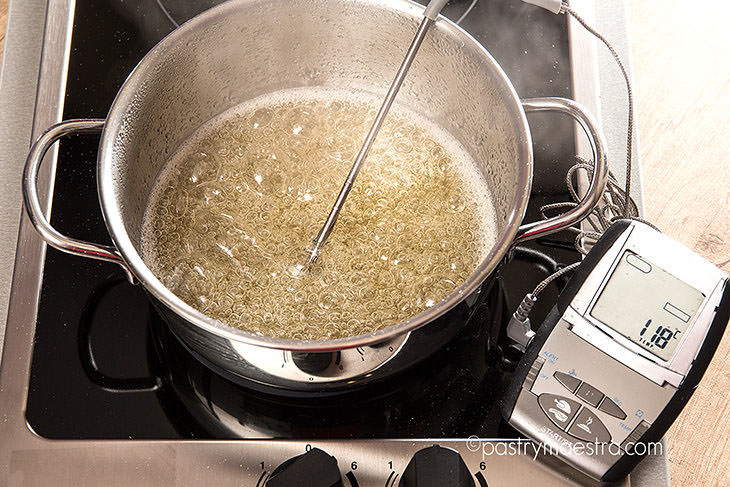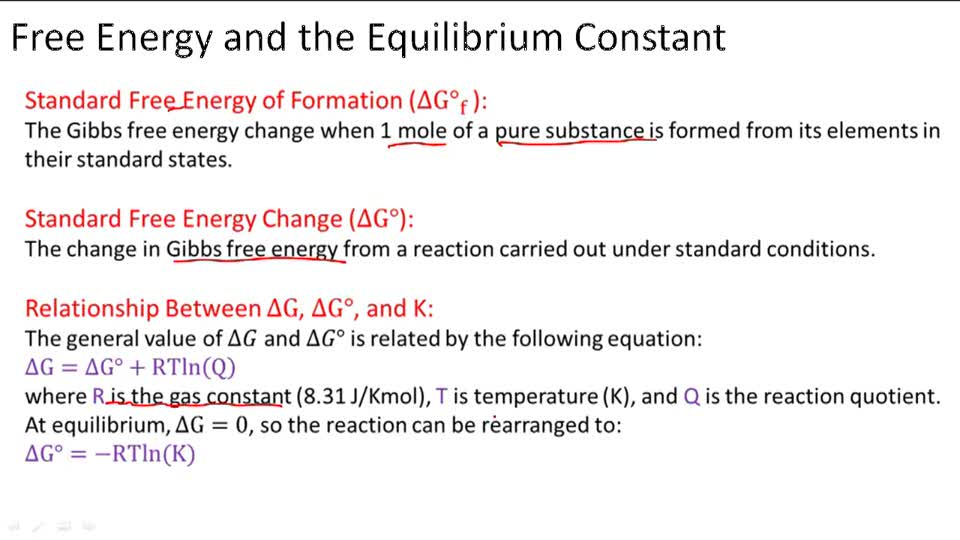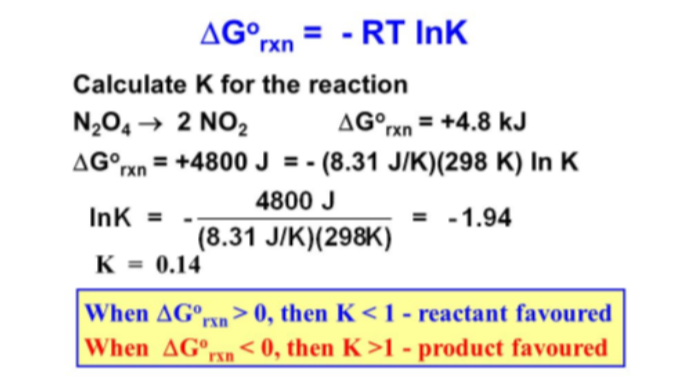Crystal

# Calculations of Free Energy and Keq Study Guide

K (equilibrium constant) and ΔG° (Gibbs free energy) can both be used to forecast the product-to-reactant ratio at equilibrium in a reaction.

Table of content

## INTRODUCTION:

You might have heard that it is not easy to make curd during the cold season! Have you ever wondered what could be the relation between room temperature and the making of curd? What could be the relation between temperature and a biological process?Source

Let's take another example to make it clear. All of us have dissolved sugar in water. Have you noticed that after dissolving a particular amount of sugar, it doesn't mix, and the sugar starts to settle down at the bottom of the container? But if you heat the water a bit, you can easily mix more sugar into the same solution! In fact, that’s how we make sugar syrup.

We keep increasing the temperature, and more and more sugar gets mixed into the solution. Have you ever wondered about the relationship between increasing temperature and the amount of sugar dissolving? The answer lies in a thermodynamic concept of spontaneity. Gibbs' free energy (ΔG°) is the concept that tells us about the spontaneity or the feasibility of any process taking place near us.Source

### EQUILIBRIUM CONSTANT (K) AND ΔG:

A reversible reaction's G is equivalent to 0 at equilibrium. The quantities of all chemicals in the process at equilibrium are referenced as Keq. As a result, we may construct the following equation (using a more sophisticated treatment of thermodynamics):Source

The computation of the other requires knowledge of either standard Gibbs free energy or the equilibrium constants for a process.

K and ΔG° can both be used to forecast the product-to-reactant ratio at equilibrium in a reaction. We have seen above that the expression that connects G° with K is ΔG°=−RTln⁡K. How do we predict the product to reactant ratio using this expression is explained below:

### LET'S LOOK AT GIBBS FREE ENERGY AND EQUILIBRIUM CONSTANT PROBLEMS:Source

## CONCLUSION:

• The quantities of all chemicals in the process at equilibrium are referenced as Keq.
• K and ΔG° can both be used to forecast the product-to-reactant ratio at equilibrium in a reaction.
• Gibb's energy and equilibrium constant have a relationship, i.e., △Go=−RTlnKeq.

### FAQs:

1. What is the relation between Gibb's free energy and equilibrium constant?

Gibb's energy and equilibrium constant have a relationship, i.e., △Go=−RTlnKeq. Gibb's energy and enthalpy change have a relationship, i.e., △G= △H- T△S.

2. How do you find the free energy from the equilibrium constant?

The equation G°=RTlnK relates G° with K.

If G° is less than zero, K is greater than one, then products are preferred over reactants at equilibrium.

At equilibrium, if G° > 0, K = 1, then reactants are preferred over products.

3. What is the Gibbs free energy at equilibrium?

The disparity here between heat radiated throughout a cycle and the heat emitted for the same set of interactions in a reversible manner is the variation in free energy (G). G = 0 indicates that a system is in equilibrium.

We hope you enjoyed studying this lesson and learned something cool about Gibbs’ free energy and equilibrium constant problems! Join our Discord community to get any questions you may have answered and to engage with other students just like you! Don't forget to download our App to experience our fun, VR classrooms - we promise, it makes studying much more fun! 😎

## SOURCES:

1. Calculations of Free Energy and Keq. https://www.ck12.org/c/chemistry/calculations-of-free-energy-and-keq/lesson/Calculations-of-Free-Energy-and-Keq-CHEM/. Accessed 28 Jan 2022.
2. Gibbs (Free) Energy. https://chem.libretexts.org/Bookshelves/Physical_and_Theoretical_Chemistry_Textbook_Maps/Supplemental_Modules_(Physical_and_Theoretical_Chemistry)/Thermodynamics/Energies_and_Potentials/Free_Energy/Gibbs_(Free)_Energy. Accessed 28 Jan 2022.
3. How do you calculate Gibbs free energy from equilibrium constant?. https://socratic.org/questions/how-do-you-calculate-gibbs-free-energy-from-equilibrium-constant. Accessed 28 Jan 2022.